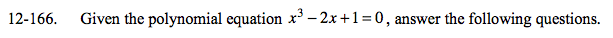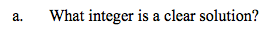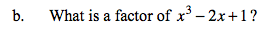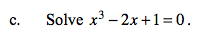### Home > A2C > Chapter Ch12 > Lesson 12.4.1 > Problem12-166

12-166.
1. Given the polynomial equation x3 − 2x + 1 = 0, answer the following questions. Homework Help ✎

1. What integer is a clear solution?

2. What is a factor of x3 − 2x + 1?

3. Solve x3 − 2x + 1 = 0.x = 1What is the factor for x = 1?If one factor is (x − 1), what is the second factor? Divide x3 − 2x + 1 by x − 1.

$x=1 \text{ or }x=\frac{-1\pm\sqrt{5}}{2}$